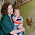## Sunday, January 31, 2016

### 32 38 44 47 93 95 122 | The Death of Kenneth L. Sailors, Denver Nuggets, who invented 'jump shot', January 30, 2016

Notice that he played for the Denver Nuggets, and this news comes a week before the Super Bowl that ones up in a lot of ways for the Denver Broncos.

the Super Bowl is on the 38th day of the year this year
Death = 4+5+1+20+8 = 38

Kenny Stills also wore the number 27 for the Denver Nuggets, and the Super Bowl is on February 7, or 2/7.  It will be Peyton Manning's 27th playoff game.  The NFC has a chance to win their 27th Super Bowl.

Nuggets = 4+3+7+7+5+2+1 = 29/38
Denver = 4+5+5+4+5+9 = 32/50 (50 with v-exeption, didn't think of that until today...)
Denver Nuggets = 71/80/89/98

Denver = 4+5+14+22+5+18 = 68
Nuggets = 14+21+7+7+5+20+19 = 93
Denver Nuggets = 161

The fact that he is remembered for the 'jump shot' definitely confirms my suspicions about his death being connected to the Super Bowl, hosted in San Francisco.

95 is the reflection of 59.  Kill sums to 59 in Jewish Gematria.  Who would be low enough to a kill a 59-year old man for the upcoming Super Bowl?

Kill = 10+9+20+20 = 59

He also attending Wyoming, the 44th State in the Union.  44 is the kill number in simple english gematria.

Kill = 11+9+12+12 = 44

Notice he played for the Cowboys in the year '42 and '43.

Cowboys = 3+6+5+2+6+7+1 = 30/39
Peyton Manning is 39
Denver is on the 39th Parallel
Charlotte = 3+8+1+9+3+6+2+2+5 = 39

Freemason = 6+9+5+5+4+1+1+6+5 = 42/51
Kenny Sailors retired in '51

http://bleacherreport.com/articles/2612509-kenny-sailors-credited-with-inventing-jump-shot-dies-at-age-95?utm_source=cnn.com&utm_medium=referral&utm_campaign=editorial

1/14/1921 = 1+14+19+21 = 55 (Broncos in 55th year of existence)
1/14/1921 = 1+14+(1+9+2+1) = 28
1/14/1921 = 1+1+4+1+9+2+1 = 19
1/14/21 = 1+14+21 = 36

1/30/2016 = 1+30+20+16 = 67 (Levi's) (Satanic) (Blood Sacrifice)
1/30/2016 = 1+30+(2+0+1+6) = 40
1/30/2016 = 1+3+0+2+0+1+6 = 13
1/30/16 = 1+30+16 = 47

Notice that he died 16-days after his birthday, and this year will be 16-years since the Broncos last won a Super Bowl, Super Bowl 33.

Sixteen = 1+9+6+2+5+5+5 = 33
Sixteen = 19+9+24+20+5+5+14 = 96 (NFL is 96-years old this year)

The first Super Bowl was in the 47th year of the NFL.

He died in the 44th state, in Laramie, WY.

Laramie, WY = 3+1+9+1+4+9+5+5+7 = 44
Kill = 11+9+12+12 = 44

Let us finish by decoding his name.

Kenneth = 2+5+5+5+5+2+8 = 32
L. = 3
Sailors = 1+1+9+3+6+9+1 = 30/39/48
Kenneth L. Sailors = 65/74/83 (Football = 83) (Masonic = 74)

Kenneth = 11+5+14+14+5+20+8 = 77 (Broncos first made playoffs in '77)
L. = 12
Sailors = 19+1+9+12+15+18+19 = 93 (Nuggets) (Crucifix) *number of sacrifice
Kenneth L. Sailors = 182

1.Jump Shot = 38 in Chaldean-Hebrew AstroNumerology. http://www.sun-angel.com/numerology/nqmulti.php

2.Zach I know you are picking Denver but you have to be completely even keel when dealing with the numbers like you are being said so the jump shot is definitely in relation to Cam Newton who celebrates by jumping in the air and shooting a jump shot love the research just consider it

1.It is a great point. Like I said in my recent video, there is a definite narrative for the Panthers. And like I said in October, if the Broncos make it, Manning and Elway are historically losers in Super Bowls. If the Panthers blew out the Broncos, it will be no surprise here. Good point on jump shot too, thank you

3.5 years ago I had warts, I was treated with some liquid applied to the warts they continued to grow and spread... The next 2 doctors did laser surgery to remove them. 1 year after the surgery, they grew back close to where the 1st ones were' so I was finally told it was hpv. I have had it for very long time, I contract it from my cheated boyfriend and I found out he was also infected and I end up the relationship between us. the warts was so embarrasses because it started spreading all over I have be dealing with this things for very long time the last treatment I take was About 2 years ago I applied natural treatment from Dr onokun herbal cure, a week after applying the treatment all the warts was gone. it's now 2 years and some months I don't have single wart or any symptoms of hpv. wow"" it's great, Dr onokun has finally cured me. Anyone living with hpv contact Dr onokun for natural treatment.
4.